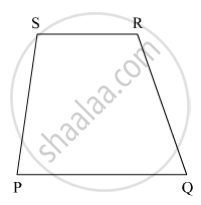SSC (English Medium) Class 8Maharashtra State Board
Share

# Ratio of Consecutive Angles of a Quadrilateral is 1:2:3:4. Find the Measure of Its Each Angle. Write, with Reason, What Type of a Quadrilateral It Is. - SSC (English Medium) Class 8 - Mathematics

ConceptKinds of Quadrilaterals Concept of Parallelogram

#### Question

Ratio of consecutive angles of a quadrilateral is 1:2:3:4. Find the measure of its each angle. Write, with reason, what type of a quadrilateral it is.

#### SolutionLet m∠P : m∠Q : m∠R : m∠S = 1 : 2 : 3 : 4

So, m∠P = k, m∠Q = 2k, m∠R = 3k and m∠S = 4k, where k is some constant

Now,

m∠P + m∠Q  + m∠R + m∠S = 360º

∴ k + 2k + 3k + 4k = 360º

⇒ 10k = 360º

⇒ k = 36º

∴ m∠P = 36º

m∠Q = 2k = 2 × 36º = 72º

m∠R = 3k = 3 × 36º = 108º

m∠S = 4k = 4 × 36º = 144º

Now,  m∠P + m∠S = 36º + 144º = 180º

We know if two lines are intersected by a transversal such that the sum of interior angles on the same of the transversal are supplementary, then the two lines are parallel.

∴ Side PQ || Side SR

Also, m∠P + m∠Q = 36º + 72º = 108º ≠ 180º

So, side PS is not parallel to side QR.

In quadrilateral PQRS, only one pair of opposite sides is parallel. Therefore, quadrilateral PQRS is a trapezium.

Is there an error in this question or solution?

#### APPEARS IN

Balbharati Solution for Balbharati Class 8 Mathematics (2019 to Current)
Chapter 8: Quadrilateral : Constructions and Types
Practice Set 8.3 | Q: 4 | Page no. 50
Solution Ratio of Consecutive Angles of a Quadrilateral is 1:2:3:4. Find the Measure of Its Each Angle. Write, with Reason, What Type of a Quadrilateral It Is. Concept: Kinds of Quadrilaterals - Concept of Parallelogram.
S